## Sample Test Problems

1. * We have shown that the Hermitian conjugate of a rotation operator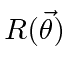is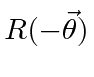. Use this to prove that if theform an orthonormal complete set, then the setare also orthonormal and complete.

2. Given that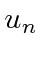is the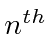one dimensional harmonic oscillator energy eigenstate: a) Evaluate the matrix element. b) Write the upper left 5 by 5 part of thematrix.

3. A spin 1 system is in the following state in the usualbasis:. What is the probability that a measurement of thecomponent of spin yields zero? What is the probability that a measurement of thecomponent of spin yields?

4. In a three state system, the matrix elements are given as,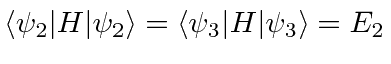,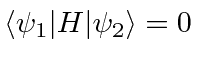,, and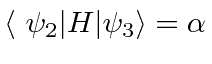. Assume all of the matrix elements are real. What are the energy eigenvalues and eigenstates of the system? Atthe system is in the state. What is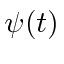?

5. Find the (normalized) eigenvectors and eigenvalues of the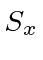(matrix) operator for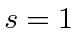in the usual () basis.

6. * A spinparticle is in a magnetic field in thedirection giving a Hamiltonian. Find the time development (matrix) operator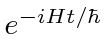in the usual basis. If, find.

7. A spinsystem is in the following state in the usualbasis: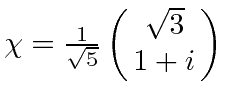. What is the probability that a measurement of thecomponent of spin yields?

8. A spinsystem is in the state(in the usualeigenstate basis). What is the probability that a measurement ofyields? What is the probability that a measurement of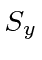yields?

9. A spinobject is in an eigenstate ofwith eigenvalueat t=0. The particle is in a magnetic field=which makes the Hamiltonian for the system. Find the probability to measure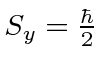as a function of time.

10. Two degenerate eigenfunctions of the Hamiltonian are properly normalized and have the following properties.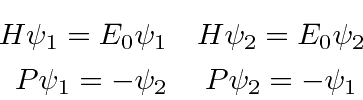What are the properly normalized states that are eigenfunctions of H and P? What are their energies?

11. What are the eigenvectors and eigenvalues for the spinoperator?

12. A spinobject is in an eigenstate ofwith eigenvalueat t=0. The particle is in a magnetic field=which makes the Hamiltonian for the system. Find the probability to measureas a function of time.

13. A spin 1 system is in the following state, (in the usualeigenstate basis):What is the probability that a measurement of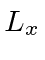yields 0? What is the probability that a measurement ofyields?

14. A spinobject is in an eigenstate ofwith eigenvalueat t=0. The particle is in a magnetic field=which makes the Hamiltonian for the system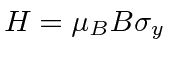. Find the probability to measureas a function of time.

15. A spin 1 particle is placed in an external field in thedirection such that the Hamiltonian is given byFind the energy eigenstates and eigenvalues.

16. A (spin) electron is in an eigenstate ofwith eigenvalue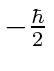at. The particle is in a magnetic field=which makes the Hamiltonian for the system. Find the spin state of the particle as a function of time. Find the probability to measureas a function of time.

Jim Branson 2013-04-22